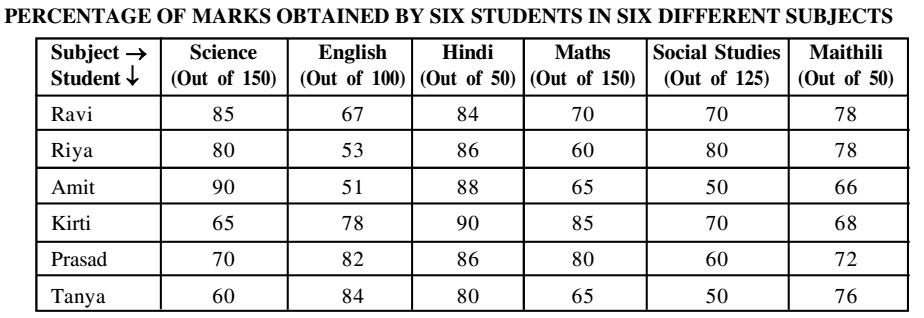Home » Data Interpretation » Table chart » Question

#### Data Interpretation

Direction: Study the table carefully to answer the questions that follow:1. If to pass in the examination the minimum marks rewired in Maths are 95 and in Social studies are 85, how many students will pass ?
1. One
2. Two
3. Three
4. Four
5. None of these
##### Correct Option: E

Pass percentage in Maths = Minimum passing marks in Maths x 100/total maths marks for math
Pass percentage in Maths = 95 x 100/150 = 63.3%
Pass percentage in Social studies = Minimum passing marks in Social studies x 100/total maths marks for Social studies
Pass percentage in Social studies = 85 x 100/125 = 68%
It is cleans from the table that Ravi and and Kirti will pass in the examination.